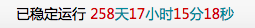# wordpress 显示网站运行天数

WP教程评论8962阅读模式PHP代码

```<span class="running-days">网站已稳定运行 <span class="blue;" id="htmer_time"></span></span>
```

```<script type="text/javascript">
/**
* Author:夏日阳光
* Author URL:https://www.pieruo.com
* Description:计算网站运行天数，本方法对闰年进行了处理
*/
function calculateDiffTime (){
var start_time = '2018/09/09 00:00:00'; // 网站开通时间
var fisrtDay = "2021/01/01 00:00:00";//用来计算闰年数，分两种情况，当前时间小于2021年和当前时间大于2021年（因为本站的建站时间为2018年，2018年后的第一个闰年是2020年，故2020年是一个分界线）

var sDate = new Date(start_time); // 开始时间
var eDate = new Date(); // 结束时间
var sY  = sDate.getFullYear();
var sM  = sDate.getMonth()+1;
var sD  = sDate.getDate();
var eY  = eDate.getFullYear();
var eM  = eDate.getMonth()+1;
var eD  = eDate.getDate();
// ------------------------------
//计算出相差年数
var years = (eY >= sY && eM >= sM && eD >= sD) ? (eY - sY) : (eY - sY - 1);
// 计算出相差天数
var rns = (eDate > new Date(fisrtDay)) ? (Math.floor(years / 4) + 1) : 0; // 计算闰年数，从2021年1月1日算起，因2020年是闰年，没有计算在内，所以总数要加1
var level = eDate.getTime() - sDate.getTime() - (rns + years * 365) * 24 * 60 * 60 * 1000; //计算年数后剩余的毫秒数，闰年按366天计
var days = Math.floor(level/(24*3600*1000));
// 计算出相差小时数
var leave1 = level % (24*3600*1000); // 计算天数后剩余的毫秒数
var hours = Math.floor(leave1/(3600*1000));
// 计算相差分钟数
var leave2 = leave1 % (3600*1000); // 计算小时数后剩余的毫秒数
var minutes = Math.floor(leave2/(60*1000));
// 计算相差秒数
var leave3 = leave2 % (60*1000); // 计算分钟数后剩余的毫秒数
var seconds = Math.round(leave3/1000);

hours = hours < 10 ? ('0' + hours) : hours;
minutes = minutes < 10 ? ('0' + minutes) : minutes;
seconds = seconds < 10 ? ('0'+seconds) : seconds;

var result = "<font class="red"> "+days + " </font>天<font class="red"> "+ hours + " </font>小时<font class="red"> " + minutes + " </font>分<font class="red"> " + seconds + " </font>秒";
result = (years > 0 ) ? (" <font class="red"> "+years + " </font>" + "年" + result) : result;
document.getElementById("htmer_time").innerHTML = currentTimeHtml;
}
setInterval(calculateDiffTime, 1000);
</script>
```

CSS美化样式：

```.footer .running-days .blue {
color: #2F889A;
}
.footer .running-days .red {
color: #C40000;
}
```• 本文由 发表于 2019年2月26日 07:52:28
• 本文为夏日阳光原创文章，转载请务必保留本文链接：https://www.pieruo.com/37.html